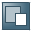Show TOC

###Variance/Covariance Approach for CFaR

The variance/covariance approach is an analytical procedure for calculating Cash Flow at Risk. The approach is based on an assumption about the distribution of risk factor changes. The variance/covariance approach is used to calculate potential losses owing to the volatility of risk factors. The volatility of the risk factors and their correlations can be estimated using the historical market data for the relevant risk factors (statistics calculator) or transferred from external sources (datafeed, market data file).

The single risks achieved in this way are aggregated using correlation matrixes under the consideration of any interference of combined effects.

Delta Valuation:

The delta approach is based on the assumption of the normal distribution of risk factor changes, and cash flow changes are estimated via the elasticity of the CF function on the different risk factors (delta positions). CF changes are also distributed using normal distribution. The quantile sought can be determined easily using the inverse values of the cumulated normal distribution function. CFaR is then achieved as the product of the quantile and the variance of the cash flow changes.

Delta/Gamma Approach:

The assumption of normal distribution does not apply. The delta/gamma approach operates in the same way as the delta approach but, in addition, includes the non-linear terms of the second order (gamma positions) at the risk factor level. In this way, skewed distributions are also interpreted. CFaR is calculated from the four moments of the distribution (expected value, variance, skewness, and kurtosis) using Cornish/Fisher approximation. It calculates the percentile of a normal distribution for a specified confidence level and for the first four moments of a distribution. The calculation of CFaR then occurs in the same way as for the delta procedure.

Delta valuation and the delta/gamma approach are also referred to as risk factor mapping.

#### Prerequisites

For the calculation of Cash Flow at Risk using the variance/covariance approach, volatilities and correlations of risk factors are required. These can be determined from historical price changes or imported to the system from third-party vendors.

In addition to calculation by the system using the statistics calculator, the RiskMetrics™ data record from JP Morgan can be imported using a file interface or a datafeed.

During the calculation, the variances and correlations are estimated for a certain holding period using the statistics calculator. For CFaR calculations using the variance/covariance approach, the variances are always read first for a holding period of one day and then scaled using the root T-method. Here, T is the period from the evaluation date until to middle of the relevant maturity band period.

#### Features

• Cash Flow at Risk for a Risk Factor

The system determines the CFaR for a risk factor by calculating the value change of the cash flow that occurs with an isolated price change of this risk factor.

This value change is calculated by determining the delta position in the risk factor and multiplying it by the standard deviation of the risk factor.

The sign (+/-) of the Cash Flow at Risk for risk factors is the same as the sign of the delta.

• Cash Flow at Risk on Hierarchy Levels

Cash Flow at Risk is a non-additive key figure and is recalculated on each level.